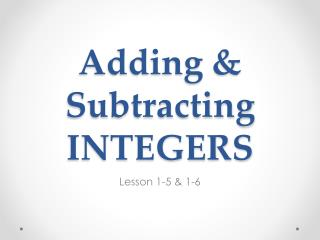DownloadDownload PresentationTélécharger la présentation- - - - - - - - - - - - - - - - - - - - - - - - - - - E N D - - - - - - - - - - - - - - - - - - - - - - - - - - -
Presentation Transcript

1. Adding & SubtractingINTEGERS Lesson 1-5 & 1-6

2. Adding & SubtractingINTEGERS • ADDING INTEGERS Same sign, add & Keep! Different sign, subtract… BUT take the sign of the higher number, then you’ll be exact

3. ADDING INTEGERS • Notice the signs… what do we do? 2 + (-5)… = -1 + 4… = 7 + (-3)… = (-2) + (-2)… =

4. Subtracting (the adding)INTEGERS • SUBTRACTING INTEGERS Negative upon negative, changes the signs. Negative and positive, creates one negative line. You’ve set up the problem, you should be glad. Now it’s even easier. Just follow the poem to add.

5. SUBTRACTING INTEGERS • Let’s change the problems to be easier (to addition): -7 – (-2)… = -4 – (-3)… = 4 – (+6)… = 5 – 9… =

6. Practice • 2 + (-6) e) 32 – (-3) b) -5 + (-1) f) -2 – 48 c) -22 + (-16) g) -8 – (-5) d) -125 + 35 h) - 40 – 66 j) -12 + (-6) + 15 + (-2)

7. Homework p. 26 #21-32 all AND p. 31 #20-34 even